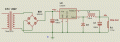# Switching supply transformer rating and IO current relation

#### alexan_e

Joined Aug 8, 2010
10
Hello

I have a question related to supply using switching regulator.
Suppose that we have a transformer rated at 1A , we are using a full wave bridge and a capacitor and suppose that the rectified output is 12v DC.

If I use a switching regulator (like LM2576 or LM2676 etc) but with an efficiency of 100% (imaginary for this example) and set the output to 6v with a 3 ohm resistor connected to the output as load (for 2A load current) then will this operate?

I would expect the regulator to work with a 50% duty to provide 6v output but this 50% would require 12v/2A pulses and the transformer can only give 1Amp so what will happen?

Is the transformer able to give pulses of higher current that the rated one as long as the average current is 1A (like 50% 2A or 25% 4A) or will all this current be provided from the bridge capacitor and the transformer will never give more than 1A?
Should the bridge capacitor be calculated for 2A instead of 1A in this case?

This is the schematic of the circuit I refer too but with a theoretical 100% efficiencyThank you
Alex

#### crutschow

Joined Mar 14, 2008
30,455
The peak transformer current will be significantly higher than 1A due to C3, thus the I$$^{2}$$R heating of the transformer will be higher than for a 1A RMS current and the transformer may overheat. So you should use a transformer rated for more than 1A. A 50% derating (1.5A transformer) should be more than sufficient.

•alexan_e

#### alexan_e

Joined Aug 8, 2010
10

How should I calculate the input transformer current ?
In the previous example you have used 3/4 of the output current so if my output current was 3A I would have to use 3/4 * 3A = 2.25A

How is this related to the input voltage , the switching regulator duty ratio lowers as the input voltage gets higher but should I keep this current I/O ratio at all times.

I was expecting to be able to use say a 30V/1A input and get 5v/3A but it doesn't seem possible from your description and the higher input voltage seems to be just a waste.

Alex

#### crutschow

Joined Mar 14, 2008
30,455
If you have 5V @ 3A output that's 15W. If you have a 30V @ 1A input capability, that's 30W. So the transformer is derated by 50% (ignoring any converter inefficiencies) which should be fine.

You use power to determine the relative input/output rating

•alexan_e

#### alexan_e

Joined Aug 8, 2010
10
Oh.. I was confused with what you said in the previous post but I think you did the calculations for a circuit with the real efficiency which is about 70% for LM2576 instead of the theoretical 100% that I was using in order to understand the operation of such circuit.

So in the schematic I have posted before if we kept the 1A input rating and increased the voltage to a higher level like for example 18v it would work fine just like the 12v/1.5A you have suggested?

What about the bridge capacitor value, I suppose we should calculate it based on the output current of the load , right?

Thank you again
Alex

#### bountyhunter

Joined Sep 7, 2009
2,512

How should I calculate the input transformer current ?
It's actually extremely complicated because the current conducts in narrow, high amplitude pulses used to be called haversine pulses. For 60Hz transformer into FWB, the approximation I always used was that the RMS winding current in the transformer is about 1.6 - 1.8X the DC load current, where that is the average current flowing into the input of the switcher regulator. Add whatever is appropriate for power loss in the regulator.

Your transformer is much too small on current for this application. You have 12W load power, add 20% for losses and get about 14W. The input to the switcher will be about 11 - 12V, so input current will be about 1.2A ballpark. Your transformer current rating would have to be at least 2A RMS.

•alexan_e

#### alexan_e

Joined Aug 8, 2010
10
The transformer rating I have used was just an example, the purpose of this post was for me to understand the operation principle of the input/output current to see if it was possible to use some of the unused transformers I have (pulled from devices) like 28v/1A to get an output of 5v/3A or 3v3/3A
.
I was hoping that the high current would stay in the path between the bridge capacitor and the output with the capacitor working as an energy storage to provide the high current pulses but from what you say the same output current will go through the transformer too and will damage it.

So if I understand correctly you are saying that I have to use a transformer having the current rating of the load or I will be in trouble , right?

Alex